# NCERT Solutions for Class 10 Maths Exercise 8.3## myCBSEguide App

Complete Guide for CBSE Students

NCERT Solutions, NCERT Exemplars, Revison Notes, Free Videos, CBSE Papers, MCQ Tests & more.

NCERT solutions for Maths Introduction to Trigonometry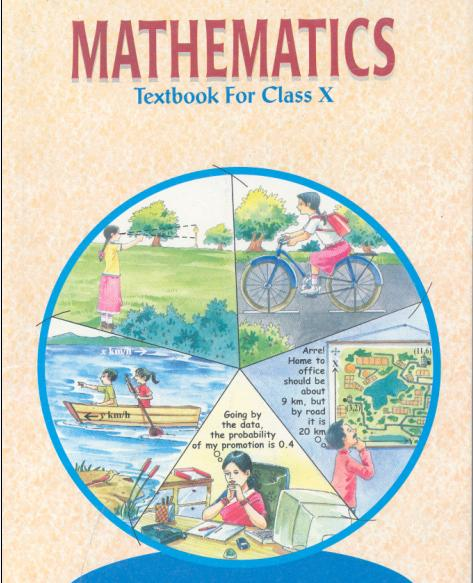## NCERT Solutions for Class 10 Maths Introduction to Trigonometry

1. Evaluate:

(i)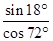(ii)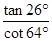(iii)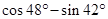(iv)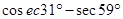Ans. (i)=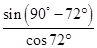=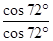= 1

(ii)=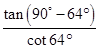=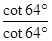= 1

(iii)=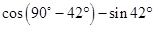=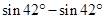= 0

(iv)=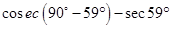=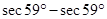=0

2. Show that:

(i)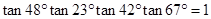(ii)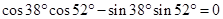Ans. (i)L.H.S.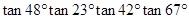=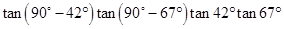=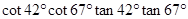=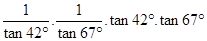= 1 = R.H.S.

(ii) R.H.S.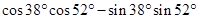=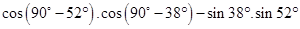=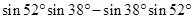= 0 = R.H.S.

3. If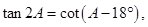where 2A is an acute angle, find the value of A.

Ans. Given: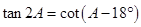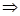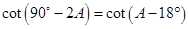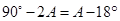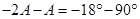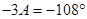A =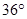4. If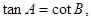prove that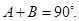Ans. Given: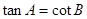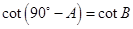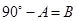A + B =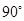5. If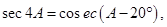where 4A is an acute angle, find the value of A.

Ans. Given: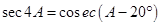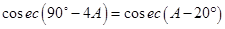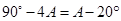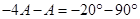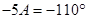A =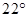6. If A, B and C are interior angles of a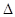ABC, then show that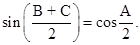Ans. Given: A, B and C are interior angles of aABC.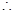A + B + C =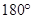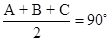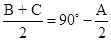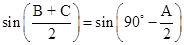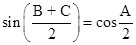7. Express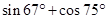in terms of trigonometric ratios of angles between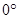and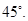Ans.=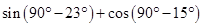=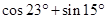## NCERT Solutions for Class 10 Maths Exercise 8.3

NCERT Solutions Class 10 Maths PDF (Download) Free from myCBSEguide app and myCBSEguide website. Ncert solution class 10 Maths includes text book solutions from Mathematics Book. NCERT Solutions for CBSE Class 10 Maths have total 15 chapters. 10 Maths NCERT Solutions in PDF for free Download on our website. Ncert Maths class 10 solutions PDF and Maths ncert class 10 PDF solutions with latest modifications and as per the latest CBSE syllabus are only available in myCBSEguide.

## CBSE app for Class 10

To download NCERT Solutions for Class 10 Maths, Computer Science, Home Science,Hindi ,English, Social Science do check myCBSEguide app or website. myCBSEguide provides sample papers with solution, test papers for chapter-wise practice, NCERT solutions, NCERT Exemplar solutions, quick revision notes for ready reference, CBSE guess papers and CBSE important question papers. Sample Paper all are made available through the best app for CBSE## Test Generator

Create Papers with your Name & Logo

### 35 thoughts on “NCERT Solutions for Class 10 Maths Exercise 8.3”

1. Nice

2. the my cbse guid is very halp full app for me
thankyou very much ?????

3. the my cbse guid is very halp full app for me
thankyou very much ?????

4. Tq for giving solutions

5. Thankyou very much ………

6. Good solutions

7. My cbse guide is very dry helpful thanx a lot

8. Thanks for the solution

9. Very helpful thanks a lot ????

10. thanku for this solutions

11. Very nice app

12. It helped me to clear my doubts.

13. Thanks the material helps me a lot

14. Good

15. Thanks for the solutions you save me from my class teacher

16. Thankyou so much

17. Thank u for this solution thanks a lot

18. Well done

19. Thank you

20. god will help to fullfill your desires?????

21. Nice explanation

22. very nice I am Ishika khare

23. Good

24. Good

25. Thanks ?

27. Thank you very much for the solutions ???

29. good help my study

30. Thank you for giving solutions

31. Nice solution

32. Thank you very much for your help!!!!!!

33. superb! help me a lot .thanks cbseguide

34. Nice sollution

35. Í ?ìke this app.